# Number System Practice Questions for SSC Exams

Number System Practice Questions for SSC CGL, CPO, CHSL, GD, Bank and other Competitive Exams. Important question answer with solution from the previous year exam question papers.

### Number System : Question Answer

Q.1: What is the greatest five-digit number that is completely divisible by 8, 15, 16, 21 and 5 ?
(A) 95760
(B) 98320
(C) 99120
(D) 92680

Ans : (C) 99120
LCM of 8, 15, 16, 21, 5 = 1680
greatest five-digit number = 99999
99999/1680 = 879 Remainder
= 99999 – 879 = 99120

Q.2: When (224 – 1) is divided by 7 , the remainder is:
(A) 4
(B) 2
(C) 1
(D) 0

Ans : (D) 0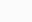$\frac{2^{24} - 1}{7}$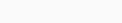$=\frac{(2^3)^8 - 1}{7}=\frac{(8)^8 - 1}{7}$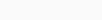$=\frac{1 - 1}{7} = \frac07 = 0$ Remanent

Q.3: If the five-digit number 672 xy is divisible by 3, 7 and 11, then what is the value of (6x + 5y) ?
(A) 16
(B) 23
(C) 17
(D) 24

Ans : (C) 17
672 xy
put x = 2, y = 1
It is divisible by 3, 7, 11
(6x + 5y)
6 x 2+5 x 1 = 12+5 = 17

Q.4: If a nine-digit number 489 x 6378 y is divisible by 72, then the value of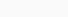$\sqrt{8x + 6y}$ will be:
(A) 10
(B) 6
(C) 8
(D) 4

Ans : (C) 8
489 x 6378 y, is divisible by 72
72 = is also divisible 9 x 8
x = 5, y = 4 satisfy
so$\sqrt{8x + 6y}$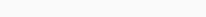$=\sqrt{8\times5 + 6\times4}=\sqrt{64}=8$

Q.5: If the nine-digit number 87605x31y is divisible by 72, then the value of 2x-3y is:
(A) -1
(B) 0
(C) 1
(D) 2

Ans : (D) 2
87605 x 31 y
is divisible by 72
Will also be divisible by 9 x 8 = 72
x = 4, y = 2 satisfy
2x – 3y
2 x 4 – 3 x 2 = 8 – 6 = 2

Q.6: When a positive integer ‘n’ is divided by 12, the remainder is 5. What will be the remainder if 8n2 + 7 is divided by 12 ?
(A) 5
(B) 3
(C) 2
(D) 4

Ans : (B) 3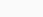$\frac {n}{12} = 5$ remainder
remainder = n = 5
8n2 + 7
8 x 52 + 7 = 207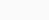$\frac{207}{12} = 3$ remainder

Q.7: If 7183 +7383 is divided by 36, the remainder is:
(A) 13
(B) 9
(C) 0
(D) 8

Ans : (C) 0
7183 +7383 factor
(71 + 73) = 144
(an+bn) = (a+b)
if n=odd No.$\frac{144}{36} = 0$ remainder

Q.8: If 3147 +4347 is divided by 37, the remainder is:
(A) 0
(B) 2
(C) 1
(D) 3

Ans : (A) 0
3147 +4347
(an+bn) = (a+b)
if n=odd No.
31+43 = 74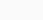$\frac{74}{37} = 0$ remainder

Q.9: If the number 4A306768B2 is divisible by both 8 and 11, then the smallest possible values of A and B will be:
(A) A = 3, B = 5
(B) A = 5, B = 4
(C) A = 5, B = 2
(D) A = 5, B = 3

Ans : (D) A = 5, B = 3
4 A 3 0 6 7 6 8 B 2
B = 3 is divisible by 8 [divisible by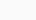$\frac{832}{8}=$ ]
The whole number is divisible by 11
A = 5
so A = 5, B = 3

Q.10: If the 11 digit number 4y6884805x6 is divisible by 72, and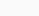$x \neq y$ , then the value of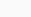$\sqrt{xy}$ is:
(A)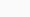$\sqrt{12}$
(B)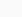$\sqrt5$
(C)$\sqrt6$
(D)$\sqrt8$

Ans : (C)$\sqrt6$
4 y 6 8 8 4 8 0 5 x 6
72 = 9×8, is divisible by 9 and 8
if x = 3$\frac{536}{8}=$ is divisible
The whole number 4 y 6 8 8 4 8 0 5 3 6 is divisible by 9.
y = 2 Keeping
The Sum of the digits will be divisible by 9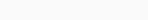$\sqrt{xy}= \sqrt{3\times2}=\sqrt6$

Q.11: When a number is divided by 3, the remainder is 2. Again, when the quotient is divided by 7, the remainder is 5. What will be the remainder when the original number is divided by 21?
(A) 14
(B) 16
(C) 13
(D) 17

Ans : (D) 17
divisor 3 7
remainder 2 5
Number (7 + 5) x 3 + 2 = 38
According of question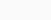$\frac{38}{21}=17$ remainder

Q.12: The largest six-digit number exactly divisible by 243 is:
(A) 999947
(B) 999945
(C) 999949
(D) 999943

Ans : (B) 999945
Largest six digit number = 999999
999999/243 = 54 remainder
= 999999 – 54 = 999945

Q.13: If the nine-digit number 259876p05 is completely divisible by 11, then what is the value of (p2 + 5) ?
(A) 48
(B) 45
(C) 54
(D) 50

Ans : (C) 54
2 5 9 8 7 6 p 0 5
is divisible by 11
Sum of digits at even places – Sum of digits at odd places = 0
(23+P) – 19 = 0 or 11
P = 7 satisfy
P2+5
72+5 = 54

Q.14: If the nine-digit number ‘8475639AB’ is divisible by 99, then what is the value of A and B ?
(A) A = 4, B = 6
(B) A = 4, B = 8
(C) A = 3, B = 9
(D) A = 5, B = 3

Ans : (B) A = 4, B = 8
8 4 7 5 6 3 9 A B,
is divisible by 99
Will also be divisible by 99 = 11 x 9
so A = 4, B = 8 satisfy
A = 4, B = 8

Thanks for attempt Number System Practice Questions for SSC CGL, CPO, CHSL, GD, Bank and other Competitive Exams.

Number System Question with Solution in Hindi for SSC Exams – SSC STUDY

## Maths Practice Set for Competitive Exams

Simple and Compound Interest Questions with Answers

Time and Work Questions with Answers

Pipe and Cistern Questions

### 2 thoughts on “Number System Practice Questions for SSC Exams”

1.yash

thankyou very much for great study material

2.sapavath akhil

it was a good session practicing a few imp logics mean while ,,,more questiond are need to provided acually

Scroll to Top